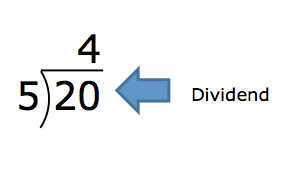Divisor

My Definition

Key Characteristics

The following are true of a divisor:

• It is the number by which the total is being separated into equal groups.

• It is the number of groups when the number in each group needs to be determined through division when using the partitive method of division.

• It is the number in each group when the number of groups needs to be determined through division when using the quotative method of division.

Example

Use the link below to further study examples of a divisor.

Divisor Activity

Non-exampleTEKS: 4(4)(F); 5(3)(C); 5(3)(F); 5(3)(G); A(10)(C)